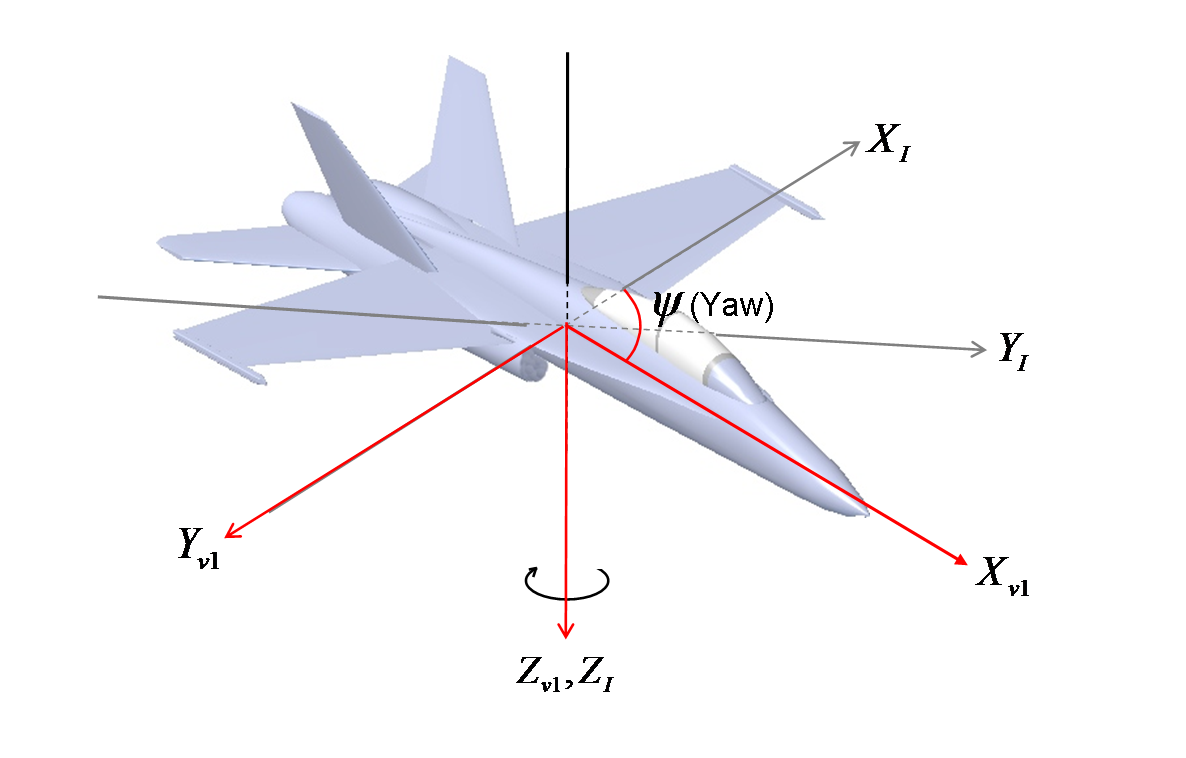### What is The Inertial Frame?

In Classic Mechanic Physics, Inertial frames are time independent, earth-fixed set of axes is used as an unmoving reference.

In my application, I’ve used FIS1100 MEMS Sensors that incorporates a 3-axis gyroscope and accelerometer also connected an external 3-axis magnetometer. As inertial frame where x-axis points east, y-axis points magnetic north pole and z-axis points along gravity.Taking into account magnetic variation I had to calculate earth-fixed coordinate from inertial frames. So there are some calculation functions in javascript.

```Calculation.eulerAngles = function(x, y, z) {
var r = Math.sqrt(Math.pow(x, 2) + Math.pow(y, 2) + Math.pow(z, 2));
var za = Math.acos(z / r);
var xa = Math.atan(y / x);
if(isNaN(za)) za = 0;
if(isNaN(xa)) xa = 0;
return {
r: r,
za: za,
xa: xa
};
}
```

Here is more detail about Euler Angles.